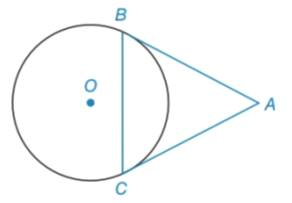Chapter 6.2, Problem 21EElementary Geometry For College St...

7th Edition
Alexander + 2 others
ISBN: 9781337614085

Solutions

Chapter
SectionElementary Geometry For College St...

7th Edition
Alexander + 2 others
ISBN: 9781337614085
Textbook Problem

In Exercises 21 and 22, complete each proof. Given: A B ¯ and A C ¯ are tangent to ⊙ o from point AProve: Δ A B C is isosceles PROOF Statements Reasons 1. ? 1. Given 2. m ∠ B = 1 2 m A B ⌢ and m ∠ C = 1 2 m B C ⌢ . 2. ? 3. m ∠ B = m ∠ C . 3. ? 4. ∠ B ≅ ∠ C . 4. ? 5. ? 5. If two ∠ s of a Δ are ≅ , the side opposite the ∠ s are ≅ . 6. ? 6. If two sides of a Δ are ≅ then Δ is isosceles.

To determine

To prove:

The given ΔABC is isosceles.

Explanation

Given:

AB¯ and AC¯ are tangent to o form point A.

Proof:

 Statements Reasons 1. AB¯ and AC¯ are tangent to ⊙o form point A. 1. Given 2. m∠B=12mAB⌢ and m∠C=12mBC⌢

Still sussing out bartleby?

Check out a sample textbook solution.

See a sample solution

The Solution to Your Study Problems

Bartleby provides explanations to thousands of textbook problems written by our experts, many with advanced degrees!

Get Started

Differentiate. f(x) = x2 sin x

Calculus: Early Transcendentals

Convert the expressions in Exercises 31-36 to positive exponent form. 12x4

Finite Mathematics and Applied Calculus (MindTap Course List)

In Exercises 1-4, simplify the expression by factoring. x23x28x7

Calculus: An Applied Approach (MindTap Course List)